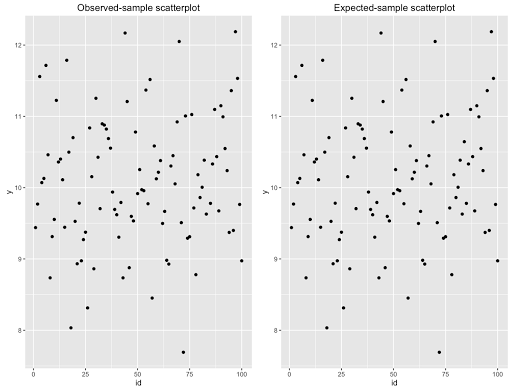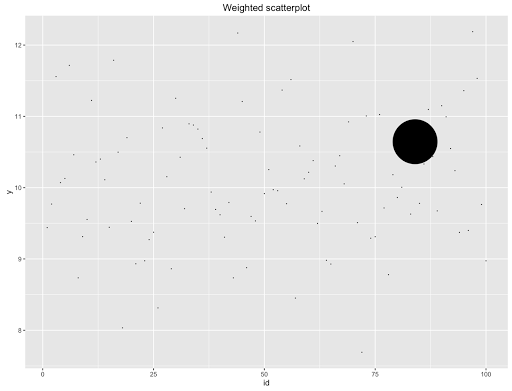Just assume that you have planned a survey along with the necessary sample size to obtain representativity. Let’s suppose the sample size is 100. However, as nonresponse is always present, unfortunately your effective sample size is 99. Consider the following figure. It shows two scatterplots, the one on the right (expected) has one more point that the one on the left (observed).Don’t they look the same? Maybe. However, if you consider sampling weights you will rapidly change your mind. You already must know that a particular unit belongs to the selected sample by means of a sampling algorithm that follows certain probability distribution defined by a vector of inclusion probabilities. Let’s suppose that the lost unit we were talking about is the one with the lowest inclusion probability. That way, that unit has the higher sampling weight. So, that single unit matters and matters a lot. Consider the following figure.The figure shows the same scatter plot. However, this time I have weighted the plot by the sampling weights of the units in the original sample. So, next time you face an issue of nonresponse think twice about ignoring it. You always have to compute how much information are you losing. An easy way to do that is by using the lost information index (LI) defined as:
$$LI = \frac{\hat{N} – \hat{N}_0}{\hat{N}}$$

Where $\hat{N}_0$ is defined as the sum of the sampling weights over the effective sample. In this specific example, we had that $\hat{N}$ = 1000, and $\hat{N}_0$ = 250. Then $LI$ = 75%.